# Selina Solutions Concise Mathematics Class 6 Chapter 32 Perimeter and Area of Plane Figures

Selina Solutions Concise Mathematics Class 6 Chapter 32 Perimeter and Area of Plane Figures contains vital formula and shortcut methods, described in simple language. The main objective of providing chapter wise solutions is to boost the exam preparation of students. Students, who want to overcome exam fear, are advised to practice these solutions on a daily basis. For enhancing problem solving skills, Selina Solutions Concise Mathematics Class 6 Chapter 32 Perimeter and Area of Plane Figures, PDF links are provided below, with a free download option.

Chapter 32 covers important concepts such as finding the perimeter and area of figures like rectangle, square and triangle. The important formulas and the method of solving problems are given in a descriptive manner, for a better conceptual knowledge among students.

## Selina Solutions Concise Mathematics Class 6 Chapter 32 Perimeter and Area of Plane Figures Download PDF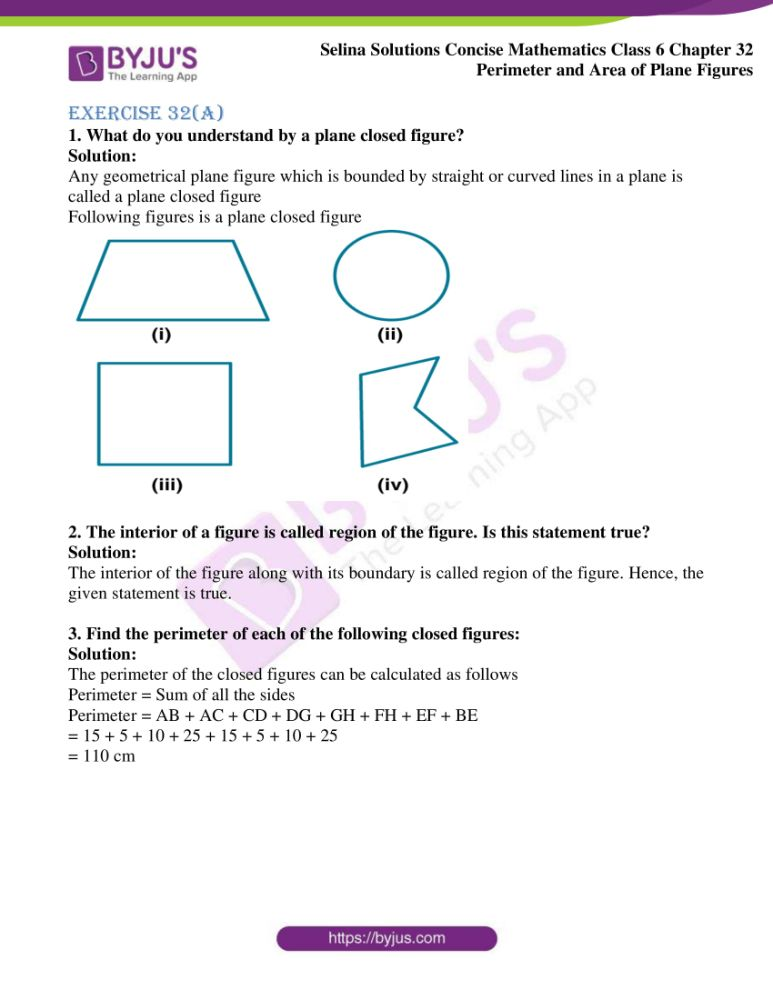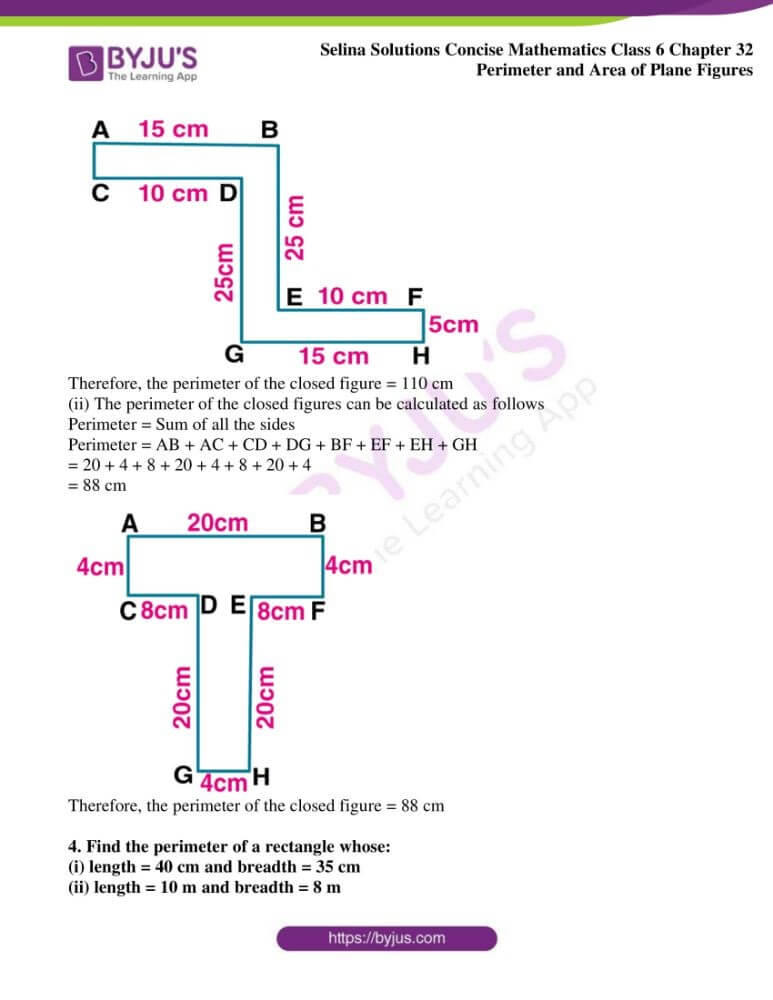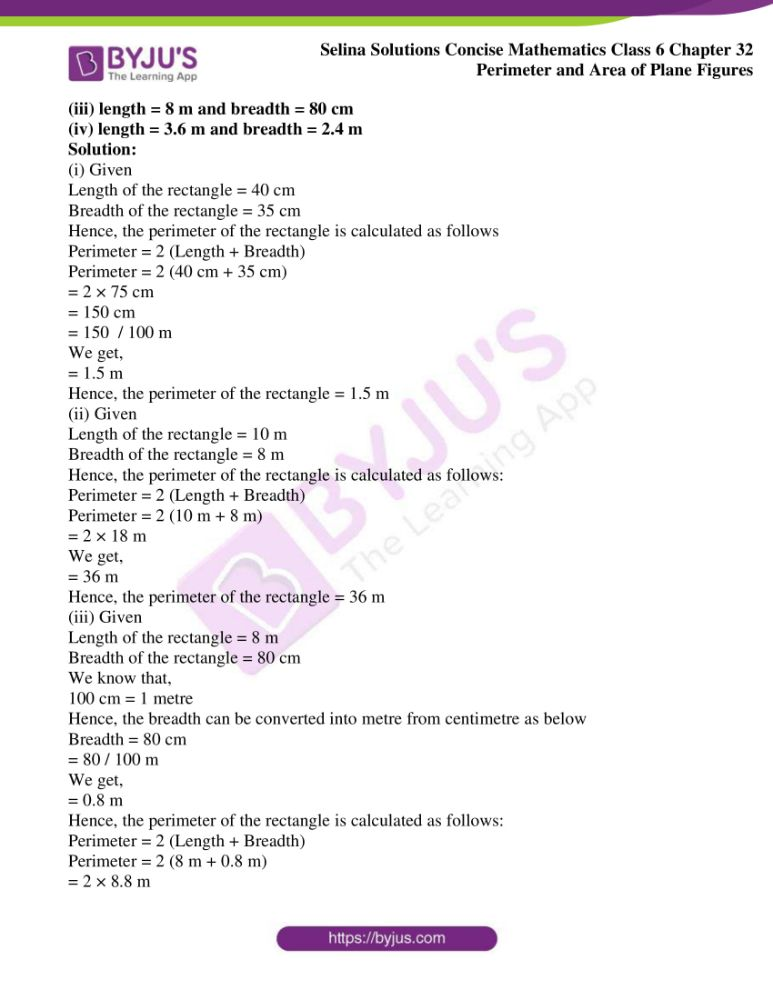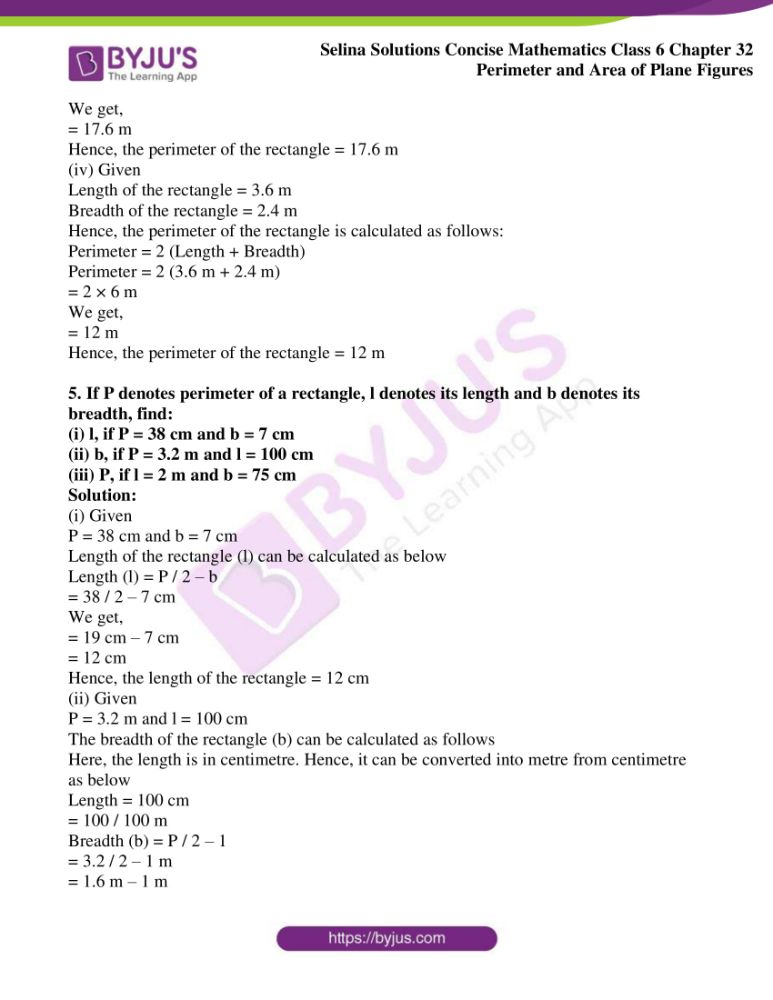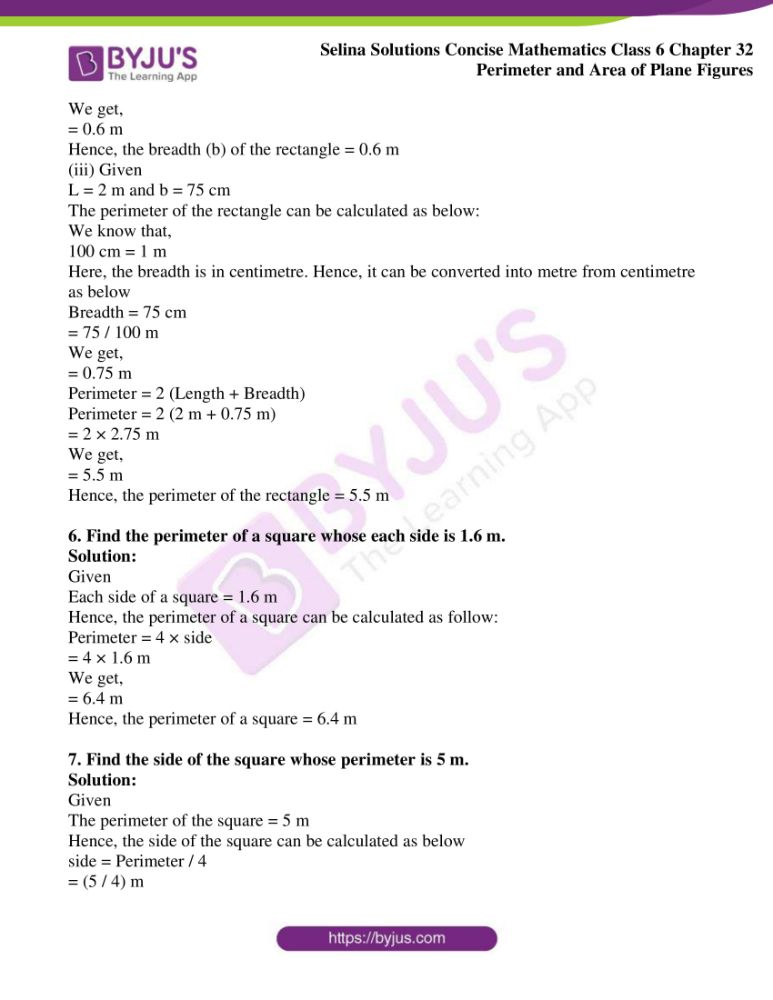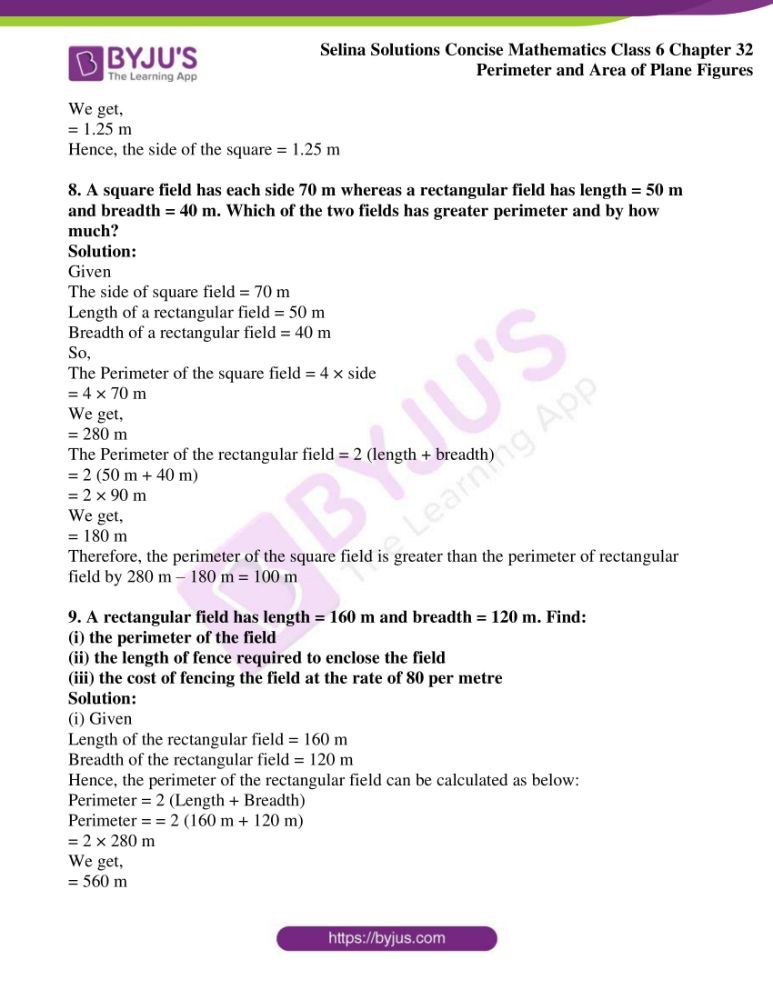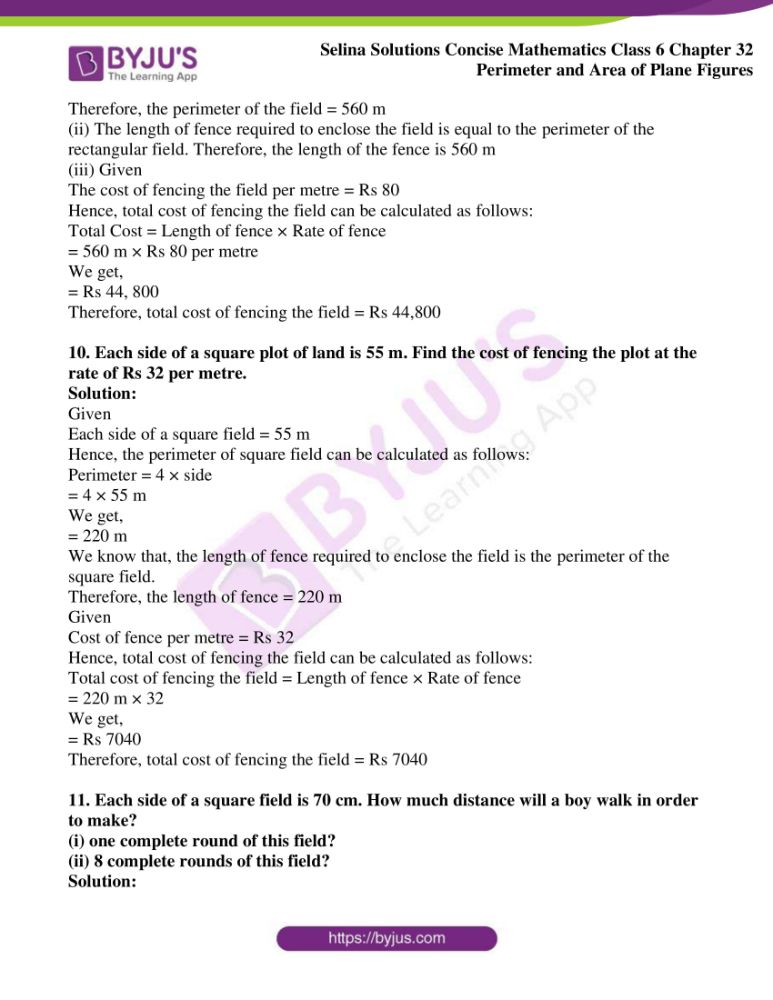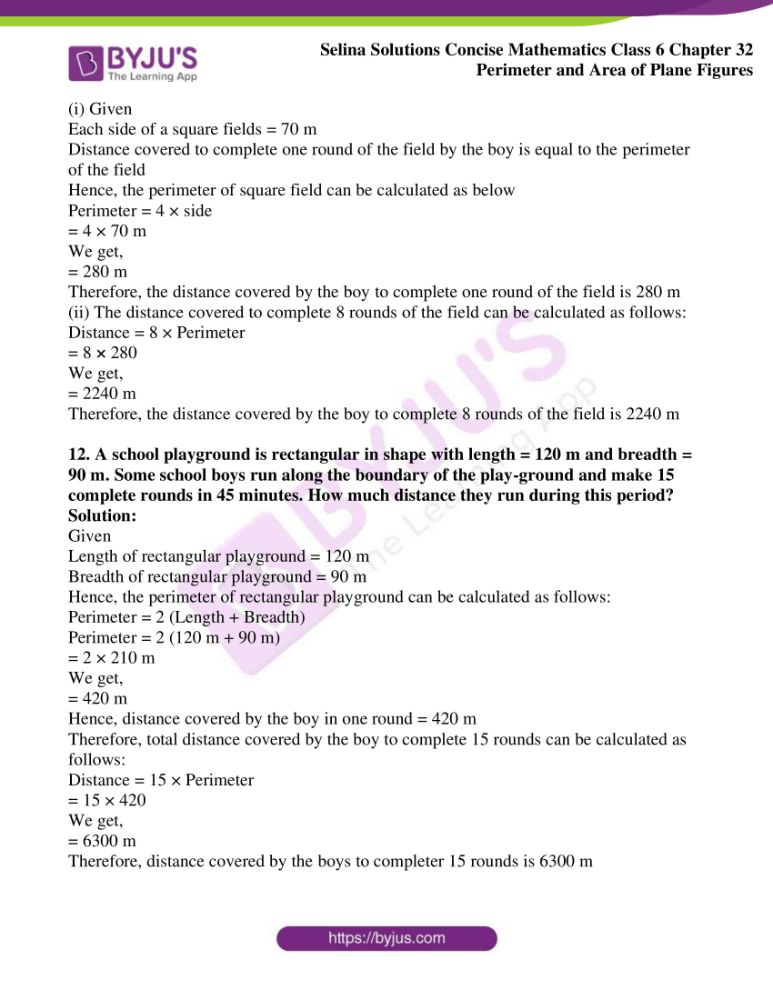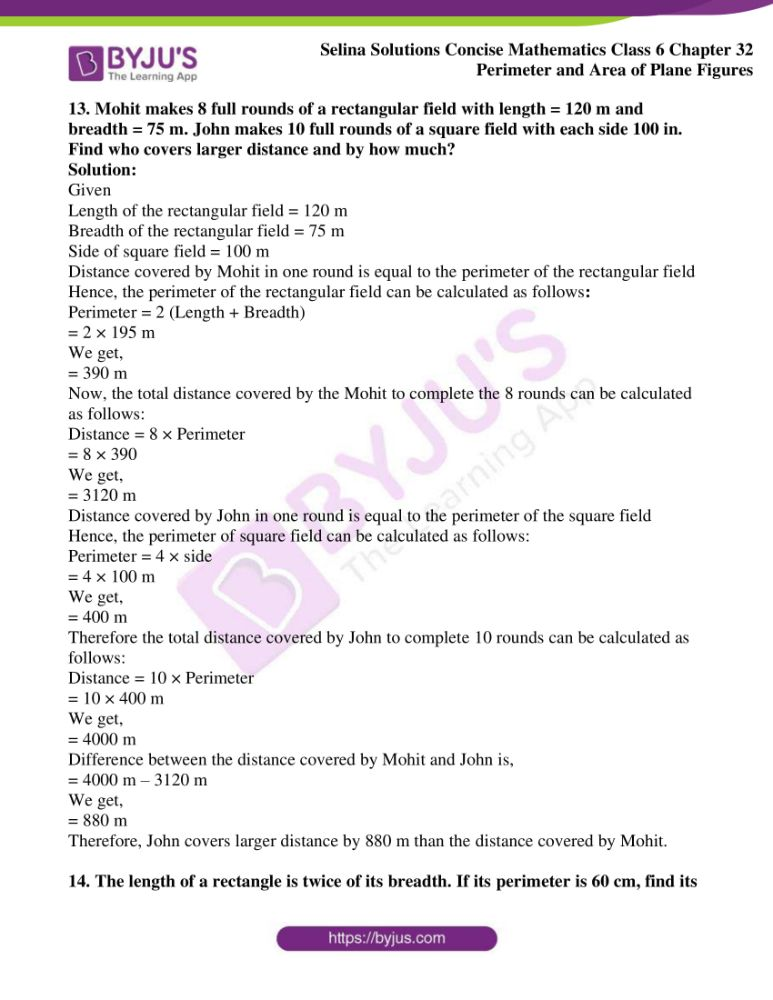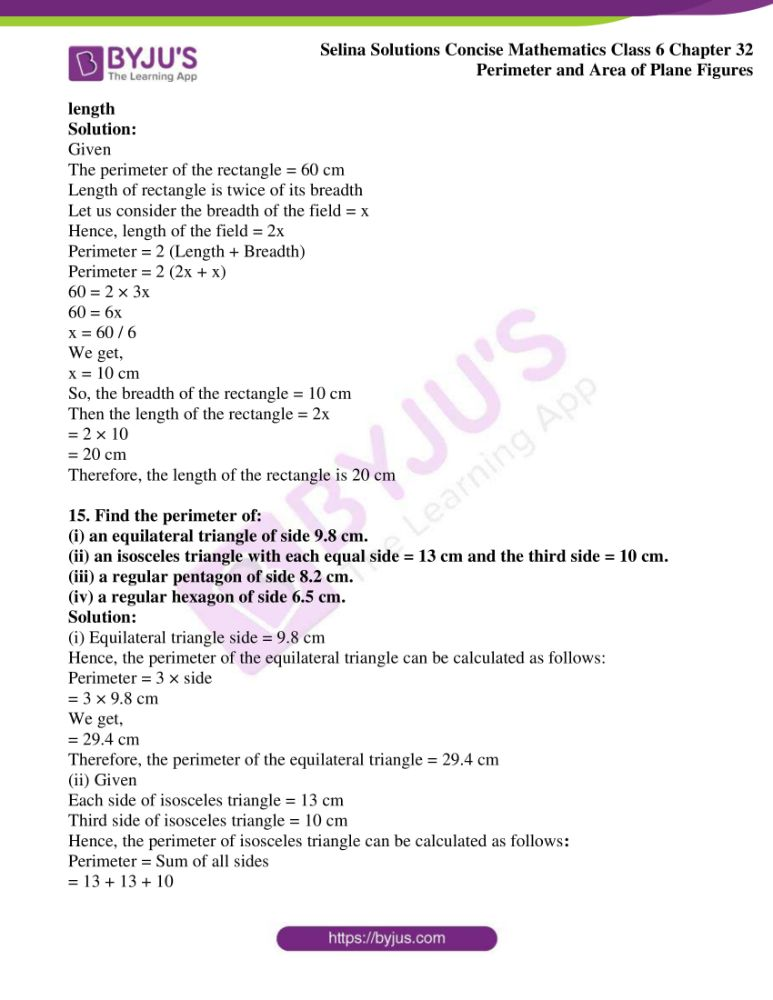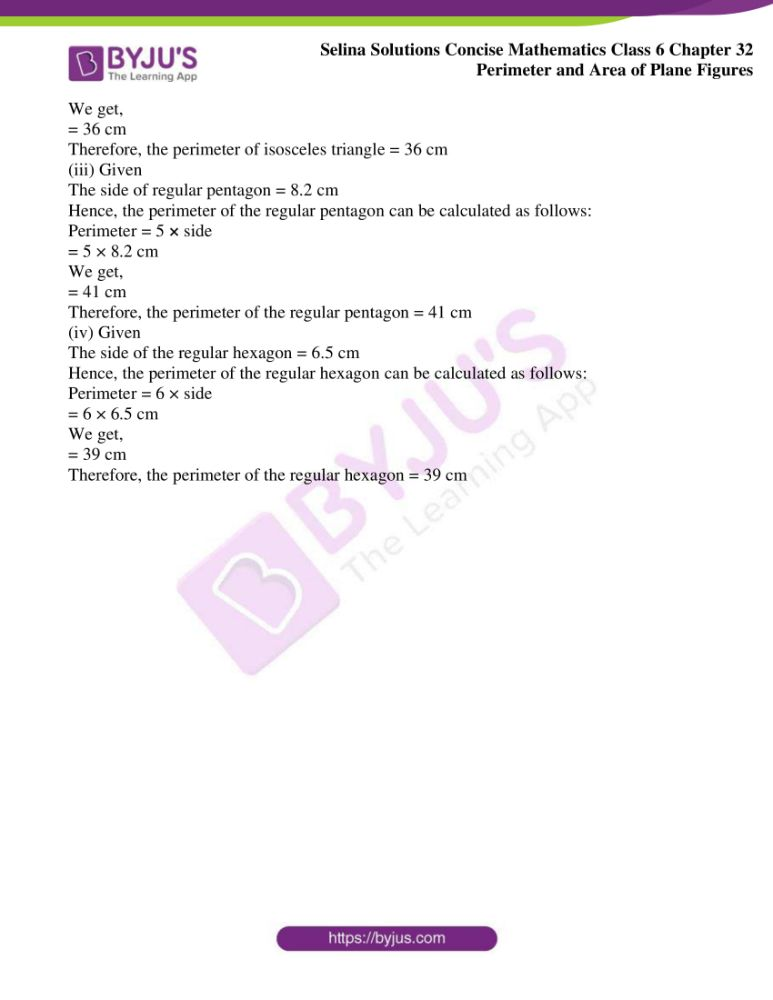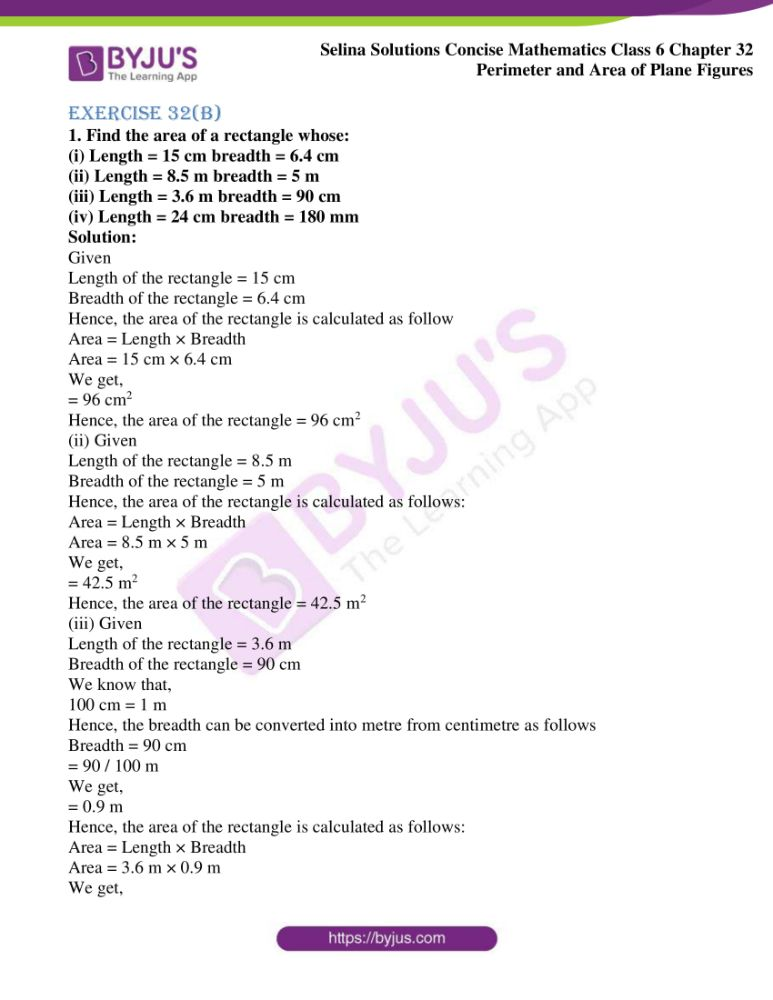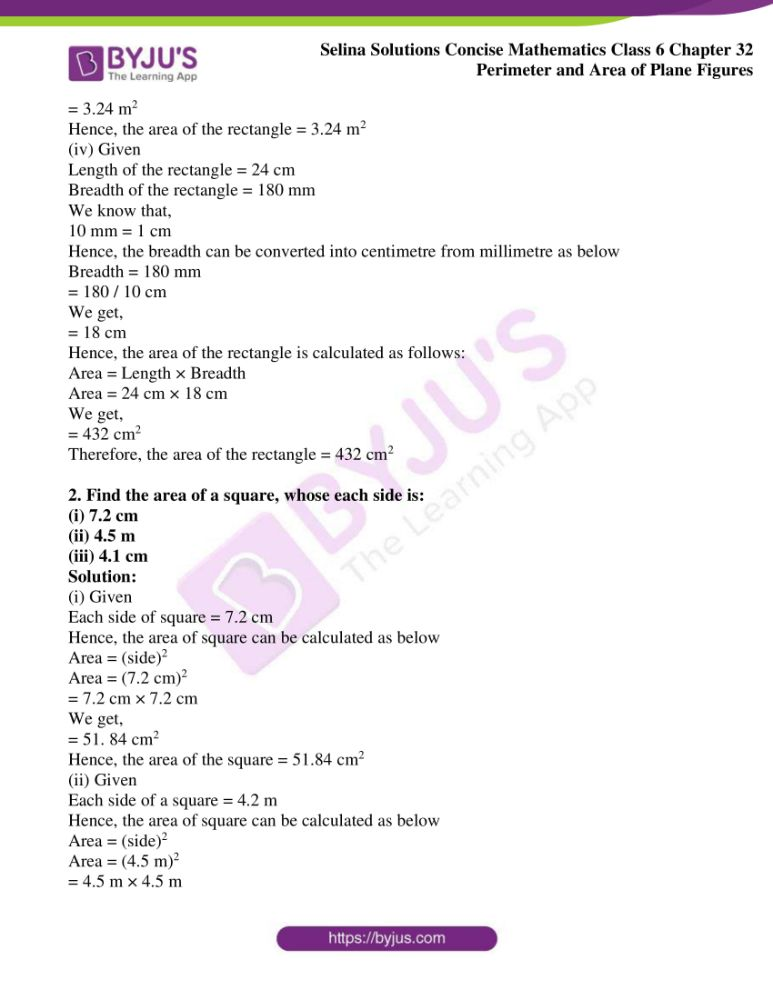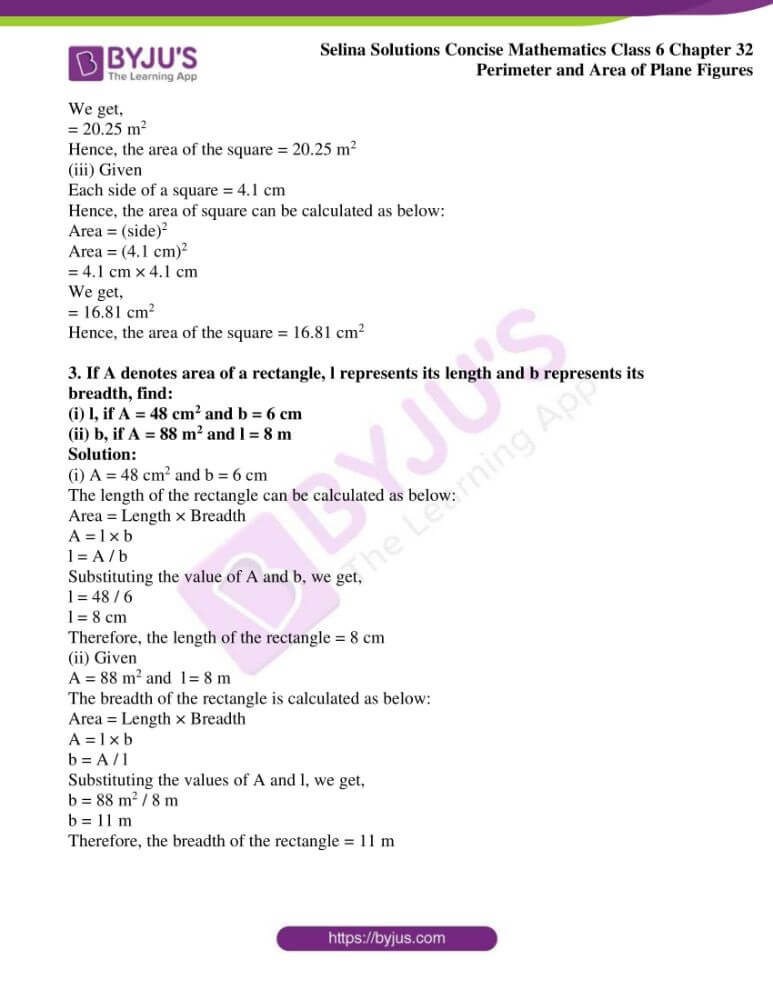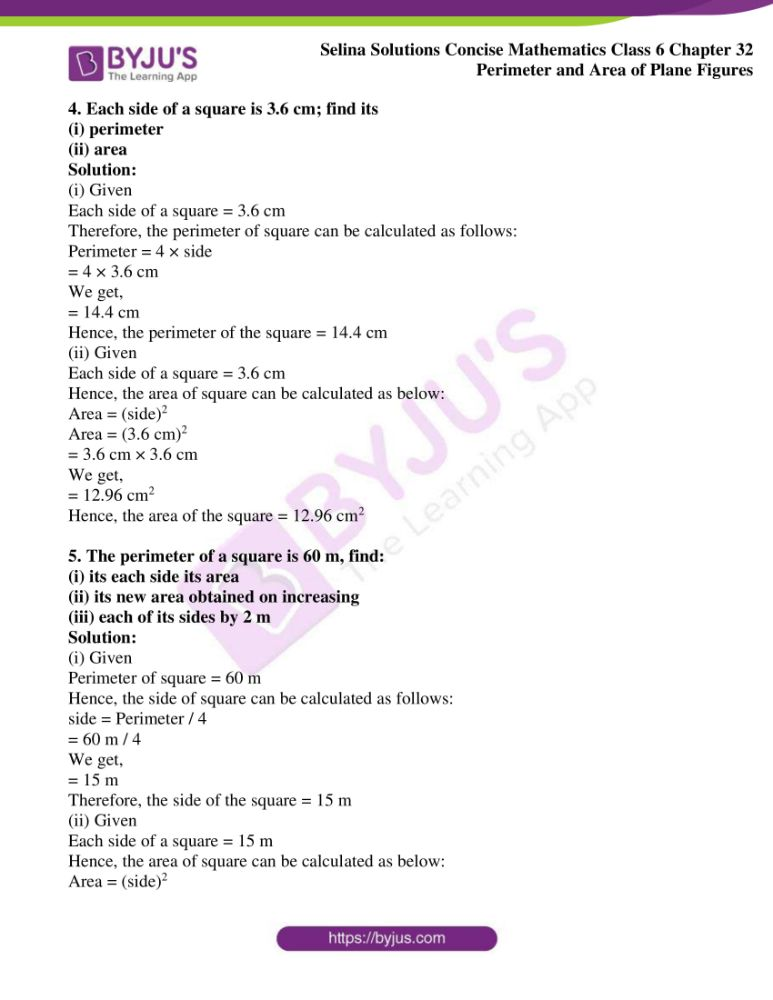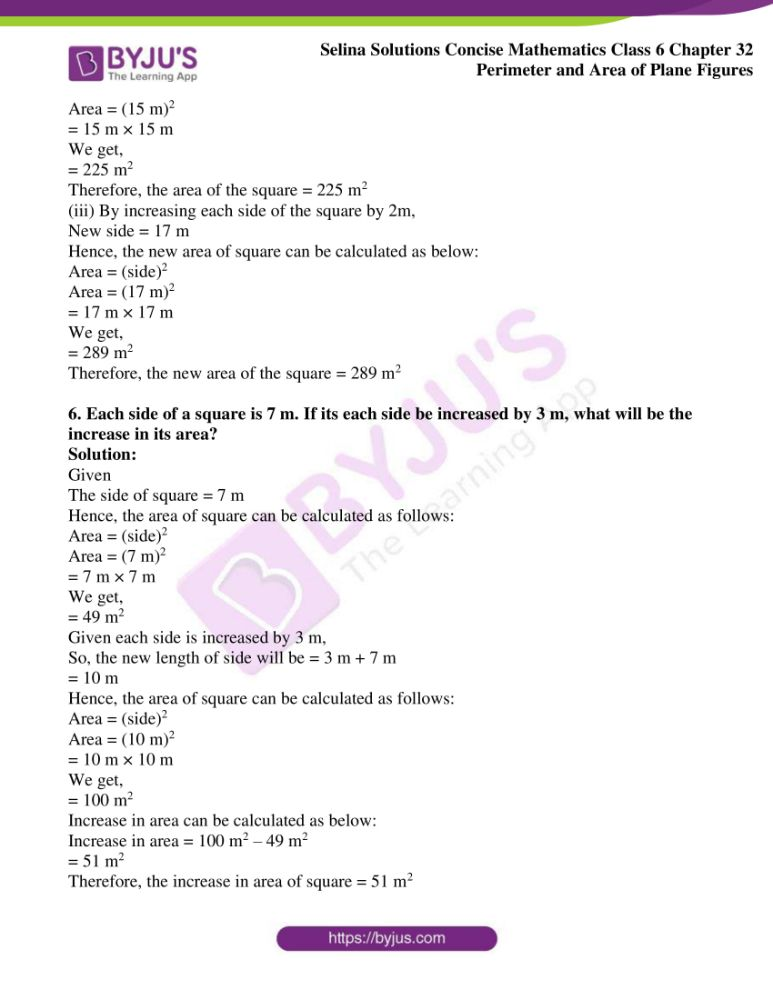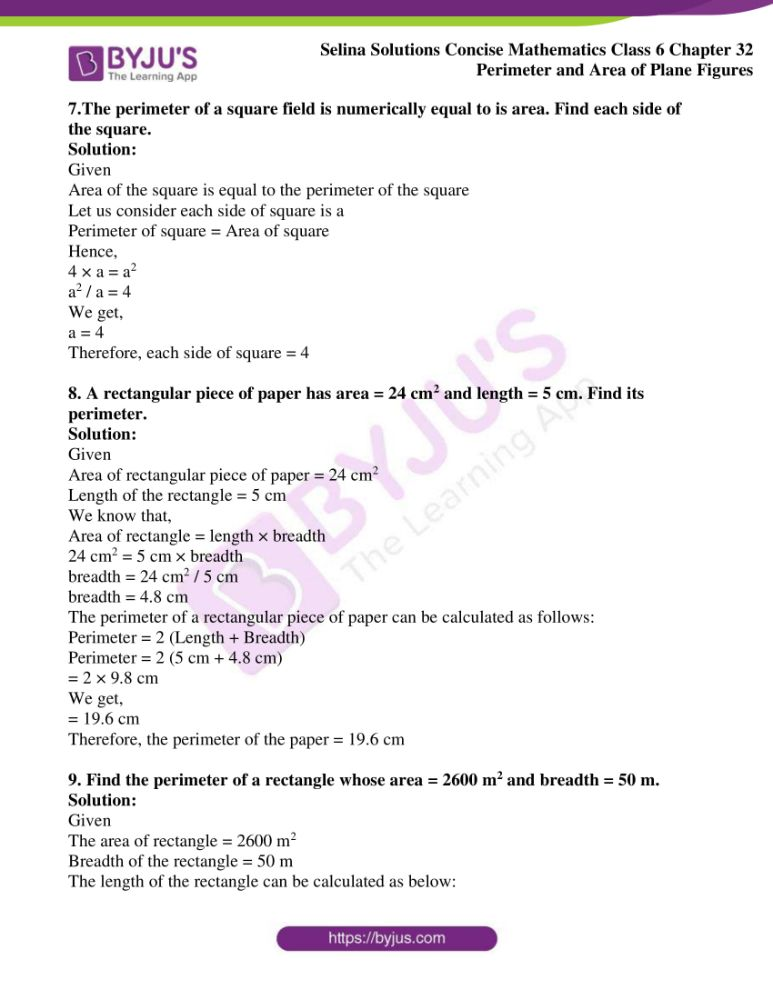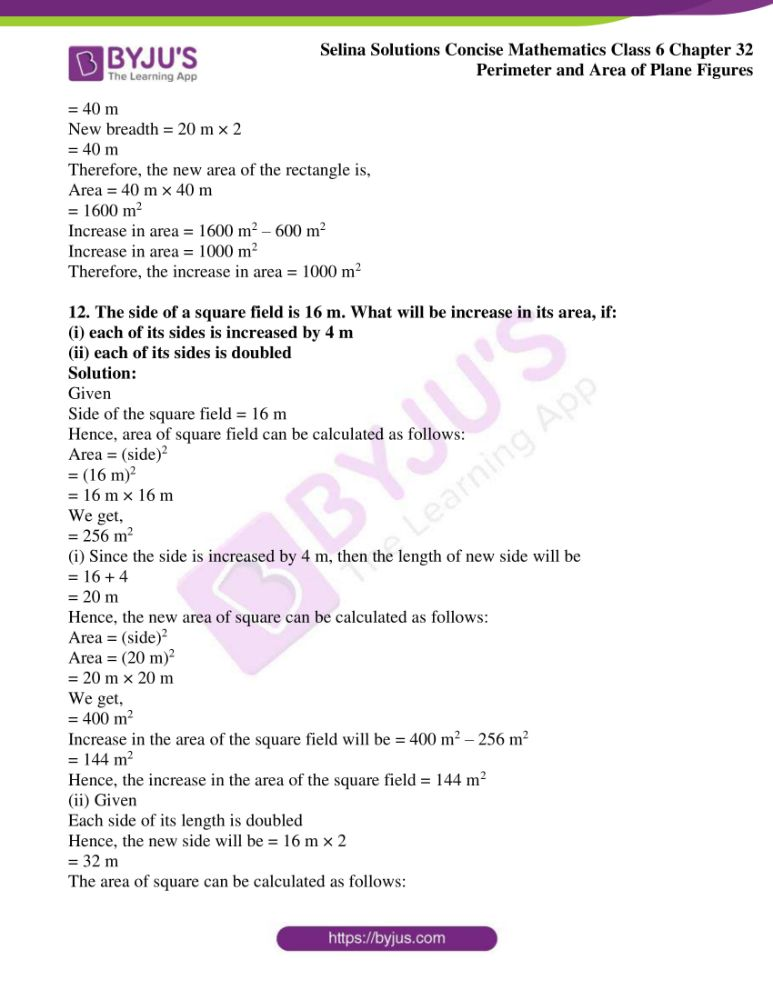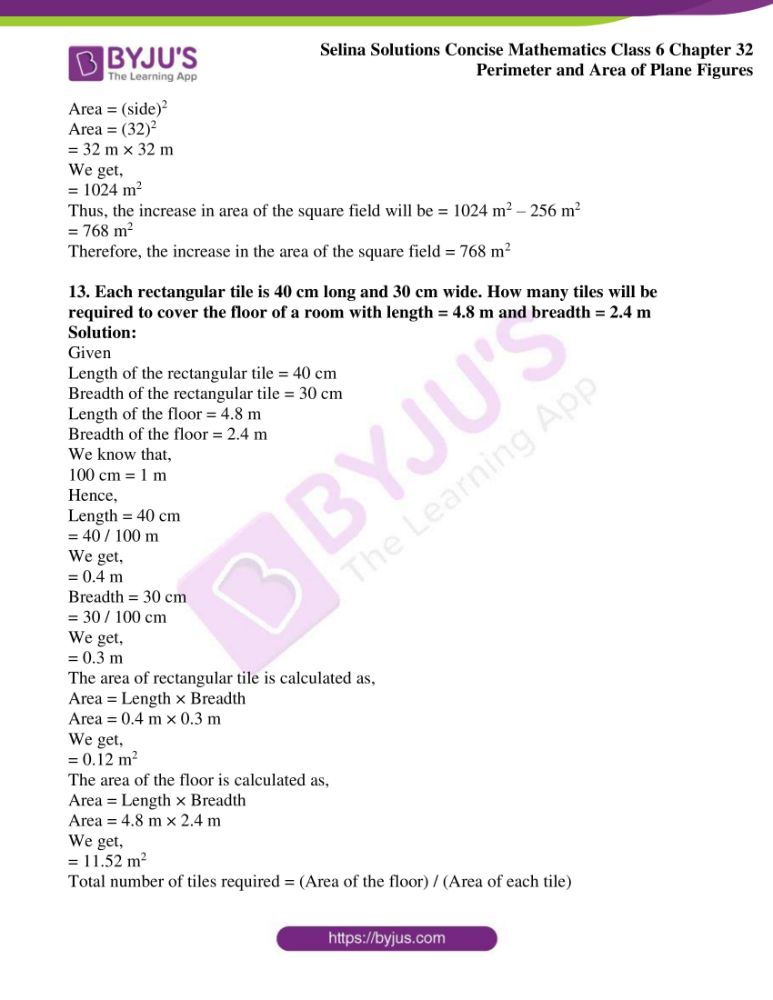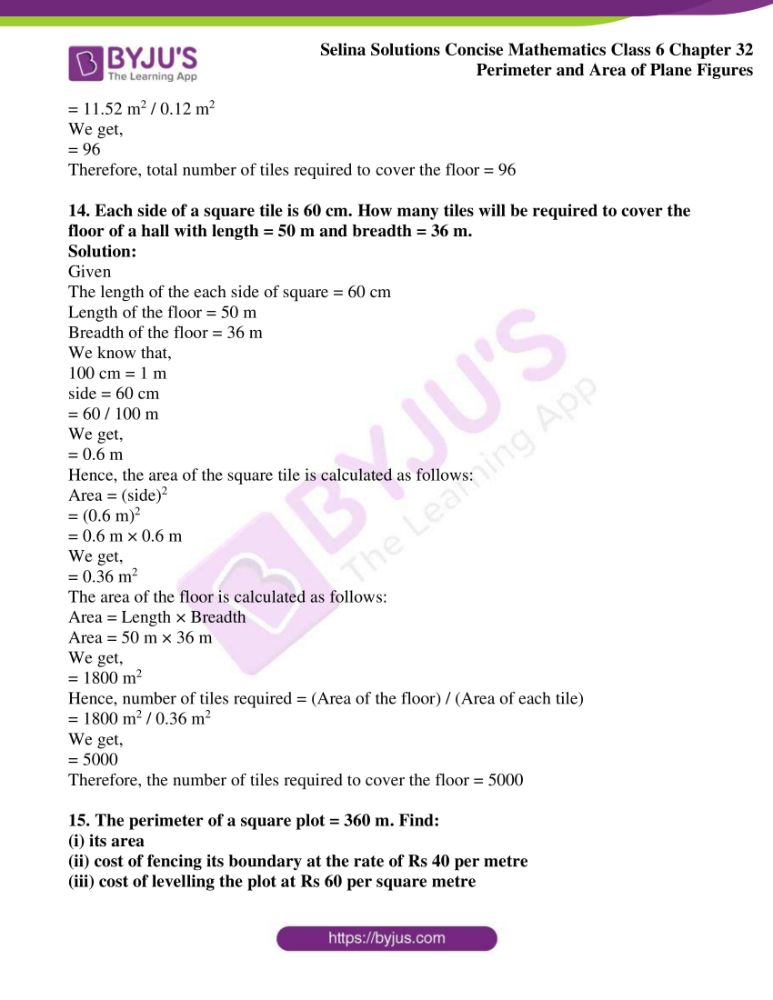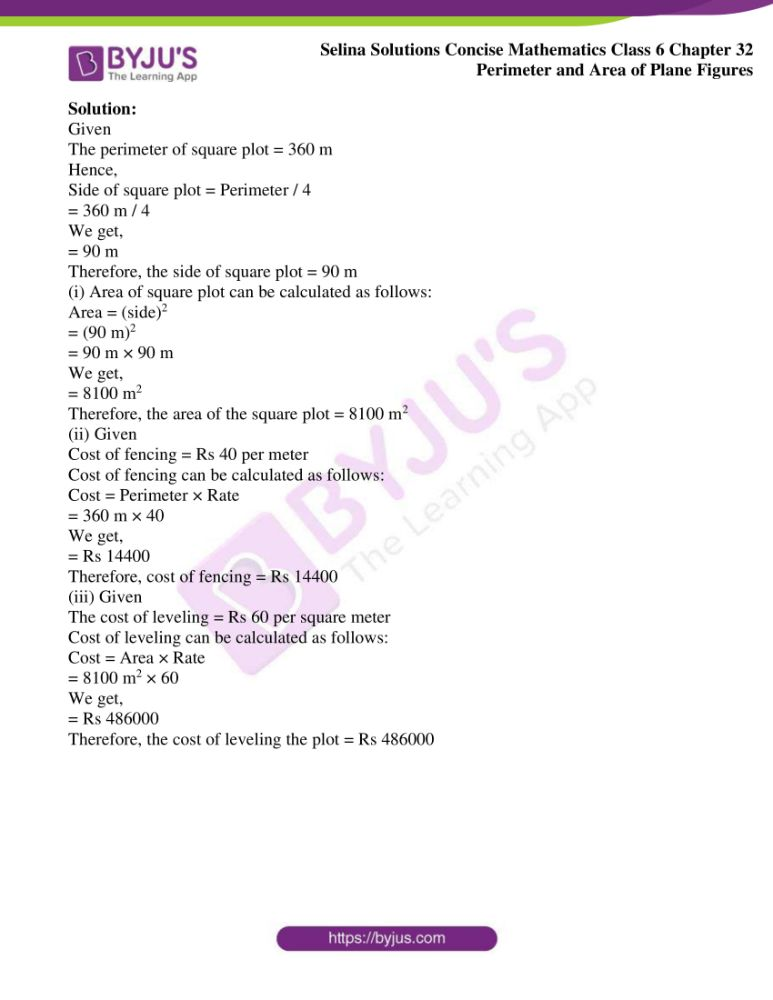### Exercises of Selina Solutions Concise Mathematics Class 6 Chapter 32 Perimeter and Area of Plane Figures

Exercise 32(A) Solutions

Exercise 32(B) Solutions

## Access Selina Solutions Concise Mathematics Class 6 Chapter 32 Perimeter and Area of Plane Figures

Exercise 32(A)

1. What do you understand by a plane closed figure?

Solution:

Any geometrical plane figure which is bounded by straight or curved lines in a plane is called a plane closed figure

Following figures is a plane closed figure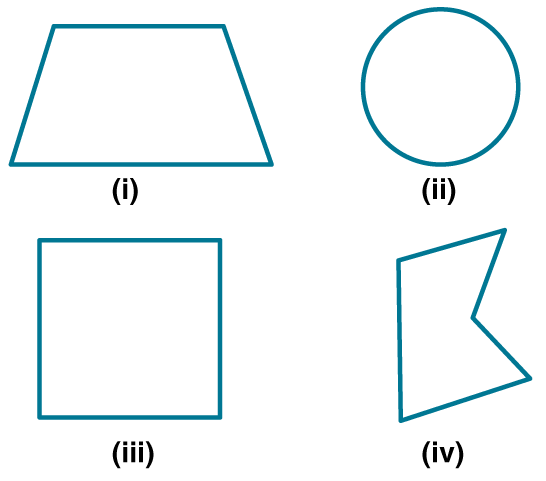2. The interior of a figure is called region of the figure. Is this statement true?

Solution:

The interior of the figure along with its boundary is called region of the figure. Hence, the given statement is true.

3. Find the perimeter of each of the following closed figures:

Solution:

The perimeter of the closed figures can be calculated as follows

Perimeter = Sum of all the sides

Perimeter = AB + AC + CD + DG + GH + FH + EF + BE

= 15 + 5 + 10 + 25 + 15 + 5 + 10 + 25

= 110 cm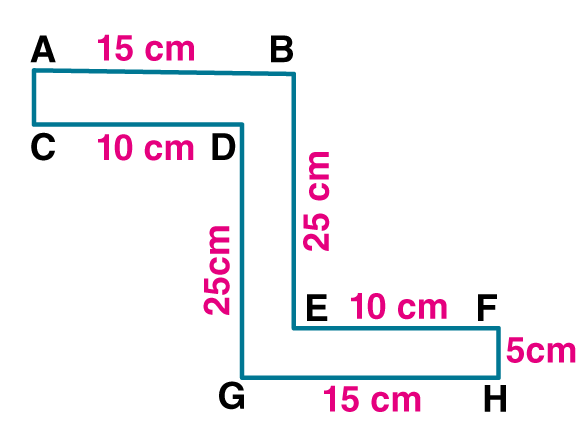Therefore, the perimeter of the closed figure = 110 cm

(ii) The perimeter of the closed figures can be calculated as follows

Perimeter = Sum of all the sides

Perimeter = AB + AC + CD + DG + BF + EF + EH + GH

= 20 + 4 + 8 + 20 + 4 + 8 + 20 + 4

= 88 cm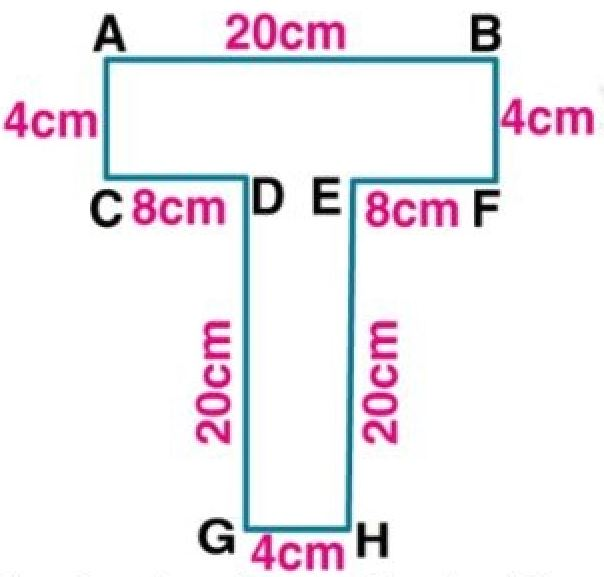Therefore, the perimeter of the closed figure = 88 cm

4. Find the perimeter of a rectangle whose:

(i) length = 40 cm and breadth = 35 cm

(ii) length = 10 m and breadth = 8 m

(iii) length = 8 m and breadth = 80 cm

(iv) length = 3.6 m and breadth = 2.4 m

Solution:

(i) Given

Length of the rectangle = 40 cm

Breadth of the rectangle = 35 cm

Hence, the perimeter of the rectangle is calculated as follows

Perimeter = 2 (Length + Breadth)

Perimeter = 2 (40 cm + 35 cm)

= 2 × 75 cm

= 150 cm

= 150 / 100 m

We get,

= 1.5 m

Hence, the perimeter of the rectangle = 1.5 m

(ii) Given

Length of the rectangle = 10 m

Breadth of the rectangle = 8 m

Hence, the perimeter of the rectangle is calculated as follows:

Perimeter = 2 (Length + Breadth)

Perimeter = 2 (10 m + 8 m)

= 2 × 18 m

We get,

= 36 m

Hence, the perimeter of the rectangle = 36 m

(iii) Given

Length of the rectangle = 8 m

Breadth of the rectangle = 80 cm

We know that,

100 cm = 1 metre

Hence, the breadth can be converted into metre from centimetre as below

= 80 / 100 m

We get,

= 0.8 m

Hence, the perimeter of the rectangle is calculated as follows:

Perimeter = 2 (Length + Breadth)

Perimeter = 2 (8 m + 0.8 m)

= 2 × 8.8 m

We get,

= 17.6 m

Hence, the perimeter of the rectangle = 17.6 m

(iv) Given

Length of the rectangle = 3.6 m

Breadth of the rectangle = 2.4 m

Hence, the perimeter of the rectangle is calculated as follows:

Perimeter = 2 (Length + Breadth)

Perimeter = 2 (3.6 m + 2.4 m)

= 2 × 6 m

We get,

= 12 m

Hence, the perimeter of the rectangle = 12 m

5. If P denotes perimeter of a rectangle, l denotes its length and b denotes its breadth, find:

(i) l, if P = 38 cm and b = 7 cm

(ii) b, if P = 3.2 m and l = 100 cm

(iii) P, if l = 2 m and b = 75 cm

Solution:

(i) Given

P = 38 cm and b = 7 cm

Length of the rectangle (l) can be calculated as below

Length (l) = P / 2 – b

= 38 / 2 – 7 cm

We get,

= 19 cm – 7 cm

= 12 cm

Hence, the length of the rectangle = 12 cm

(ii) Given

P = 3.2 m and l = 100 cm

The breadth of the rectangle (b) can be calculated as follows

Here, the length is in centimetre. Hence, it can be converted into metre from centimetre as below

Length = 100 cm

= 100 / 100 m

Breadth (b) = P / 2 – 1

= 3.2 / 2 – 1 m

= 1.6 m – 1 m

We get,

= 0.6 m

Hence, the breadth (b) of the rectangle = 0.6 m

(iii) Given

L = 2 m and b = 75 cm

The perimeter of the rectangle can be calculated as below:

We know that,

100 cm = 1 m

Here, the breadth is in centimetre. Hence, it can be converted into metre from centimetre as below

= 75 / 100 m

We get,

= 0.75 m

Perimeter = 2 (Length + Breadth)

Perimeter = 2 (2 m + 0.75 m)

= 2 × 2.75 m

We get,

= 5.5 m

Hence, the perimeter of the rectangle = 5.5 m

6. Find the perimeter of a square whose each side is 1.6 m.

Solution:

Given

Each side of a square = 1.6 m

Hence, the perimeter of a square can be calculated as follow:

Perimeter = 4 × side

= 4 × 1.6 m

We get,

= 6.4 m

Hence, the perimeter of a square = 6.4 m

7. Find the side of the square whose perimeter is 5 m.

Solution:

Given

The perimeter of the square = 5 m

Hence, the side of the square can be calculated as below

side = Perimeter / 4

= (5 / 4) m

We get,

= 1.25 m

Hence, the side of the square = 1.25 m

8. A square field has each side 70 m whereas a rectangular field has length = 50 m and breadth = 40 m. Which of the two fields has greater perimeter and by how much?

Solution:

Given

The side of square field = 70 m

Length of a rectangular field = 50 m

Breadth of a rectangular field = 40 m

So,

The Perimeter of the square field = 4 × side

= 4 × 70 m

We get,

= 280 m

The Perimeter of the rectangular field = 2 (length + breadth)

= 2 (50 m + 40 m)

= 2 × 90 m

We get,

= 180 m

Therefore, the perimeter of the square field is greater than the perimeter of rectangular field by 280 m – 180 m = 100 m

9. A rectangular field has length = 160 m and breadth = 120 m. Find:

(i) the perimeter of the field

(ii) the length of fence required to enclose the field

(iii) the cost of fencing the field at the rate of 80 per metre

Solution:

(i) Given

Length of the rectangular field = 160 m

Breadth of the rectangular field = 120 m

Hence, the perimeter of the rectangular field can be calculated as below:

Perimeter = 2 (Length + Breadth)

Perimeter = = 2 (160 m + 120 m)

= 2 × 280 m

We get,

= 560 m

Therefore, the perimeter of the field = 560 m

(ii) The length of fence required to enclose the field is equal to the perimeter of the rectangular field. Therefore, the length of the fence is 560 m

(iii) Given

The cost of fencing the field per metre = Rs 80

Hence, total cost of fencing the field can be calculated as follows:

Total Cost = Length of fence × Rate of fence

= 560 m × Rs 80 per metre

We get,

= Rs 44, 800

Therefore, total cost of fencing the field = Rs 44,800

10. Each side of a square plot of land is 55 m. Find the cost of fencing the plot at the rate of Rs 32 per metre.

Solution:

Given

Each side of a square field = 55 m

Hence, the perimeter of square field can be calculated as follows:

Perimeter = 4 × side

= 4 × 55 m

We get,

= 220 m

We know that, the length of fence required to enclose the field is the perimeter of the square field.

Therefore, the length of fence = 220 m

Given

Cost of fence per metre = Rs 32

Hence, total cost of fencing the field can be calculated as follows:

Total cost of fencing the field = Length of fence × Rate of fence

= 220 m × 32

We get,

= Rs 7040

Therefore, total cost of fencing the field = Rs 7040

11. Each side of a square field is 70 cm. How much distance will a boy walk in order to make?

(i) one complete round of this field?

(ii) 8 complete rounds of this field?

Solution:

(i) Given

Each side of a square fields = 70 m

Distance covered to complete one round of the field by the boy is equal to the perimeter of the field

Hence, the perimeter of square field can be calculated as below

Perimeter = 4 × side

= 4 × 70 m

We get,

= 280 m

Therefore, the distance covered by the boy to complete one round of the field is 280 m

(ii) The distance covered to complete 8 rounds of the field can be calculated as follows:

Distance = 8 × Perimeter

= 8 × 280

We get,

= 2240 m

Therefore, the distance covered by the boy to complete 8 rounds of the field is 2240 m

12. A school playground is rectangular in shape with length = 120 m and breadth = 90 m. Some school boys run along the boundary of the play-ground and make 15 complete rounds in 45 minutes. How much distance they run during this period?

Solution:

Given

Length of rectangular playground = 120 m

Breadth of rectangular playground = 90 m

Hence, the perimeter of rectangular playground can be calculated as follows:

Perimeter = 2 (Length + Breadth)

Perimeter = 2 (120 m + 90 m)

= 2 × 210 m

We get,

= 420 m

Hence, distance covered by the boy in one round = 420 m

Therefore, total distance covered by the boy to complete 15 rounds can be calculated as follows:

Distance = 15 × Perimeter

= 15 × 420

We get,

= 6300 m

Therefore, distance covered by the boys to completer 15 rounds is 6300 m

13. Mohit makes 8 full rounds of a rectangular field with length = 120 m and breadth = 75 m. John makes 10 full rounds of a square field with each side 100 in. Find who covers larger distance and by how much?

Solution:

Given

Length of the rectangular field = 120 m

Breadth of the rectangular field = 75 m

Side of square field = 100 m

Distance covered by Mohit in one round is equal to the perimeter of the rectangular field

Hence, the perimeter of the rectangular field can be calculated as follows:

Perimeter = 2 (Length + Breadth)

= 2 × 195 m

We get,

= 390 m

Now, the total distance covered by the Mohit to complete the 8 rounds can be calculated as follows:

Distance = 8 × Perimeter

= 8 × 390

We get,

= 3120 m

Distance covered by John in one round is equal to the perimeter of the square field

Hence, the perimeter of square field can be calculated as follows:

Perimeter = 4 × side

= 4 × 100 m

We get,

= 400 m

Therefore the total distance covered by John to complete 10 rounds can be calculated as follows:

Distance = 10 × Perimeter

= 10 × 400 m

We get,

= 4000 m

Difference between the distance covered by Mohit and John is,

= 4000 m – 3120 m

We get,

= 880 m

Therefore, John covers larger distance by 880 m than the distance covered by Mohit.

14. The length of a rectangle is twice of its breadth. If its perimeter is 60 cm, find its length

Solution:

Given

The perimeter of the rectangle = 60 cm

Length of rectangle is twice of its breadth

Let us consider the breadth of the field = x

Hence, length of the field = 2x

Perimeter = 2 (Length + Breadth)

Perimeter = 2 (2x + x)

60 = 2 × 3x

60 = 6x

x = 60 / 6

We get,

x = 10 cm

So, the breadth of the rectangle = 10 cm

Then the length of the rectangle = 2x

= 2 × 10

= 20 cm

Therefore, the length of the rectangle is 20 cm

15. Find the perimeter of:

(i) an equilateral triangle of side 9.8 cm.

(ii) an isosceles triangle with each equal side = 13 cm and the third side = 10 cm.

(iii) a regular pentagon of side 8.2 cm.

(iv) a regular hexagon of side 6.5 cm.

Solution:

(i) Equilateral triangle side = 9.8 cm

Hence, the perimeter of the equilateral triangle can be calculated as follows:

Perimeter = 3 × side

= 3 × 9.8 cm

We get,

= 29.4 cm

Therefore, the perimeter of the equilateral triangle = 29.4 cm

(ii) Given

Each side of isosceles triangle = 13 cm

Third side of isosceles triangle = 10 cm

Hence, the perimeter of isosceles triangle can be calculated as follows:

Perimeter = Sum of all sides

= 13 + 13 + 10

We get,

= 36 cm

Therefore, the perimeter of isosceles triangle = 36 cm

(iii) Given

The side of regular pentagon = 8.2 cm

Hence, the perimeter of the regular pentagon can be calculated as follows:

Perimeter = 5 × side

= 5 × 8.2 cm

We get,

= 41 cm

Therefore, the perimeter of the regular pentagon = 41 cm

(iv) Given

The side of the regular hexagon = 6.5 cm

Hence, the perimeter of the regular hexagon can be calculated as follows:

Perimeter = 6 × side

= 6 × 6.5 cm

We get,

= 39 cm

Therefore, the perimeter of the regular hexagon = 39 cm

Exercise 32(B)

1. Find the area of a rectangle whose:

(i) Length = 15 cm breadth = 6.4 cm

(ii) Length = 8.5 m breadth = 5 m

(iii) Length = 3.6 m breadth = 90 cm

(iv) Length = 24 cm breadth = 180 mm

Solution:

Given

Length of the rectangle = 15 cm

Breadth of the rectangle = 6.4 cm

Hence, the area of the rectangle is calculated as follow

Area = 15 cm × 6.4 cm

We get,

= 96 cm2

Hence, the area of the rectangle = 96 cm2

(ii) Given

Length of the rectangle = 8.5 m

Breadth of the rectangle = 5 m

Hence, the area of the rectangle is calculated as follows:

Area = 8.5 m × 5 m

We get,

= 42.5 m2

Hence, the area of the rectangle = 42.5 m2

(iii) Given

Length of the rectangle = 3.6 m

Breadth of the rectangle = 90 cm

We know that,

100 cm = 1 m

Hence, the breadth can be converted into metre from centimetre as follows

= 90 / 100 m

We get,

= 0.9 m

Hence, the area of the rectangle is calculated as follows:

Area = 3.6 m × 0.9 m

We get,

= 3.24 m2

Hence, the area of the rectangle = 3.24 m2

(iv) Given

Length of the rectangle = 24 cm

Breadth of the rectangle = 180 mm

We know that,

10 mm = 1 cm

Hence, the breadth can be converted into centimetre from millimetre as below

= 180 / 10 cm

We get,

= 18 cm

Hence, the area of the rectangle is calculated as follows:

Area = 24 cm × 18 cm

We get,

= 432 cm2

Therefore, the area of the rectangle = 432 cm2

2. Find the area of a square, whose each side is:

(i) 7.2 cm

(ii) 4.5 m

(iii) 4.1 cm

Solution:

(i) Given

Each side of square = 7.2 cm

Hence, the area of square can be calculated as below

Area = (side)2

Area = (7.2 cm)2

= 7.2 cm × 7.2 cm

We get,

= 51. 84 cm2

Hence, the area of the square = 51.84 cm2

(ii) Given

Each side of a square = 4.2 m

Hence, the area of square can be calculated as below

Area = (side)2

Area = (4.5 m)2

= 4.5 m × 4.5 m

We get,

= 20.25 m2

Hence, the area of the square = 20.25 m2

(iii) Given

Each side of a square = 4.1 cm

Hence, the area of square can be calculated as below:

Area = (side)2

Area = (4.1 cm)2

= 4.1 cm × 4.1 cm

We get,

= 16.81 cm2

Hence, the area of the square = 16.81 cm2

3. If A denotes area of a rectangle, l represents its length and b represents its breadth, find:

(i) l, if A = 48 cm2 and b = 6 cm

(ii) b, if A = 88 m2 and l = 8 m

Solution:

(i) A = 48 cm2 and b = 6 cm

The length of the rectangle can be calculated as below:

A = l × b

l = A / b

Substituting the value of A and b, we get,

l = 48 / 6

l = 8 cm

Therefore, the length of the rectangle = 8 cm

(ii) Given

A = 88 m2 and l = 8 m

The breadth of the rectangle is calculated as below:

A = l × b

b = A / l

Substituting the values of A and l, we get,

b = 88 m2 / 8 m

b = 11 m

Therefore, the breadth of the rectangle = 11 m

4. Each side of a square is 3.6 cm; find its

(i) perimeter

(ii) area

Solution:

(i) Given

Each side of a square = 3.6 cm

Therefore, the perimeter of square can be calculated as follows:

Perimeter = 4 × side

= 4 × 3.6 cm

We get,

= 14.4 cm

Hence, the perimeter of the square = 14.4 cm

(ii) Given

Each side of a square = 3.6 cm

Hence, the area of square can be calculated as below:

Area = (side)2

Area = (3.6 cm)2

= 3.6 cm × 3.6 cm

We get,

= 12.96 cm2

Hence, the area of the square = 12.96 cm2

5. The perimeter of a square is 60 m, find:

(i) its each side its area

(ii) its new area obtained on increasing

(iii) each of its sides by 2 m

Solution:

(i) Given

Perimeter of square = 60 m

Hence, the side of square can be calculated as follows:

side = Perimeter / 4

= 60 m / 4

We get,

= 15 m

Therefore, the side of the square = 15 m

(ii) Given

Each side of a square = 15 m

Hence, the area of square can be calculated as below:

Area = (side)2

Area = (15 m)2

= 15 m × 15 m

We get,

= 225 m2

Therefore, the area of the square = 225 m2

(iii) By increasing each side of the square by 2m,

New side = 17 m

Hence, the new area of square can be calculated as below:

Area = (side)2

Area = (17 m)2

= 17 m × 17 m

We get,

= 289 m2

Therefore, the new area of the square = 289 m2

6. Each side of a square is 7 m. If its each side be increased by 3 m, what will be the increase in its area?

Solution:

Given

The side of square = 7 m

Hence, the area of square can be calculated as follows:

Area = (side)2

Area = (7 m)2

= 7 m × 7 m

We get,

= 49 m2

Given each side is increased by 3 m,

So, the new length of side will be = 3 m + 7 m

= 10 m

Hence, the area of square can be calculated as follows:

Area = (side)2

Area = (10 m)2

= 10 m × 10 m

We get,

= 100 m2

Increase in area can be calculated as below:

Increase in area = 100 m2 – 49 m2

= 51 m2

Therefore, the increase in area of square = 51 m2

7.The perimeter of a square field is numerically equal to is area. Find each side of the square.

Solution:

Given

Area of the square is equal to the perimeter of the square

Let us consider each side of square is a

Perimeter of square = Area of square

Hence,

4 × a = a2

a2 / a = 4

We get,

a = 4

Therefore, each side of square = 4

8. A rectangular piece of paper has area = 24 cm2 and length = 5 cm. Find its perimeter.

Solution:

Given

Area of rectangular piece of paper = 24 cm2

Length of the rectangle = 5 cm

We know that,

Area of rectangle = length × breadth

24 cm2 = 5 cm × breadth

breadth = 24 cm2 / 5 cm

The perimeter of a rectangular piece of paper can be calculated as follows:

Perimeter = 2 (Length + Breadth)

Perimeter = 2 (5 cm + 4.8 cm)

= 2 × 9.8 cm

We get,

= 19.6 cm

Therefore, the perimeter of the paper = 19.6 cm

9. Find the perimeter of a rectangle whose area = 2600 m2 and breadth = 50 m.

Solution:

Given

The area of rectangle = 2600 m2

Breadth of the rectangle = 50 m

The length of the rectangle can be calculated as below:

= 2600 m2 / 50 m

We get,

= 52 m

Hence, the perimeter of rectangle can be calculated as below:

Perimeter = 2 (Length + Breadth)

Perimeter = 2 (52 m + 50 m)

= 2 × 102 m

We get,

= 204 m

Therefore, the perimeter of the rectangle = 204 m

10. What will happen to the area of a rectangle, if its length and breadth both are trippled?

Solution:

Let us consider the length of the rectangle is l and breadth of the rectangle is b

Since,

Length and breadth of the rectangle are trippled

So, new length and breadth will be = 3l × 3b

Hence, the new area of the rectangle is,

Area = 3l × 3b

We get,

Area = 9 × l × b

Therefore, the new area will be 9 times than the original area of the rectangle

11. Length of a rectangle is 30 m and its breadth is 20 m. Find the increase in its area if its length is increased by 10 m and its breadth is doubled.

Solution:

Given

The length of a rectangle = 30 m

The breadth of rectangle = 20 m

The area of the rectangle can be calculated as follows:

= 30 m × 20 m

= 600 m2

Also, given that the length is increased by 10 m and its breadth is doubled

Hence, the new length and breadth is as follows:

New length = 30 m + 10 m

= 40 m

New breadth = 20 m × 2

= 40 m

Therefore, the new area of the rectangle is,

Area = 40 m × 40 m

= 1600 m2

Increase in area = 1600 m2 – 600 m2

Increase in area = 1000 m2

Therefore, the increase in area = 1000 m2

12. The side of a square field is 16 m. What will be increase in its area, if:

(i) each of its sides is increased by 4 m

(ii) each of its sides is doubled

Solution:

Given

Side of the square field = 16 m

Hence, area of square field can be calculated as follows:

Area = (side)2

= (16 m)2

= 16 m × 16 m

We get,

= 256 m2

(i) Since the side is increased by 4 m, then the length of new side will be

= 16 + 4

= 20 m

Hence, the new area of square can be calculated as follows:

Area = (side)2

Area = (20 m)2

= 20 m × 20 m

We get,

= 400 m2

Increase in the area of the square field will be = 400 m2 – 256 m2

= 144 m2

Hence, the increase in the area of the square field = 144 m2

(ii) Given

Each side of its length is doubled

Hence, the new side will be = 16 m × 2

= 32 m

The area of square can be calculated as follows:

Area = (side)2

Area = (32)2

= 32 m × 32 m

We get,

= 1024 m2

Thus, the increase in area of the square field will be = 1024 m2 – 256 m2

= 768 m2

Therefore, the increase in the area of the square field = 768 m2

13. Each rectangular tile is 40 cm long and 30 cm wide. How many tiles will be required to cover the floor of a room with length = 4.8 m and breadth = 2.4 m

Solution:

Given

Length of the rectangular tile = 40 cm

Breadth of the rectangular tile = 30 cm

Length of the floor = 4.8 m

Breadth of the floor = 2.4 m

We know that,

100 cm = 1 m

Hence,

Length = 40 cm

= 40 / 100 m

We get,

= 0.4 m

= 30 / 100 cm

We get,

= 0.3 m

The area of rectangular tile is calculated as,

Area = 0.4 m × 0.3 m

We get,

= 0.12 m2

The area of the floor is calculated as,

Area = 4.8 m × 2.4 m

We get,

= 11.52 m2

Total number of tiles required = (Area of the floor) / (Area of each tile)

= 11.52 m2 / 0.12 m2

We get,

= 96

Therefore, total number of tiles required to cover the floor = 96

14. Each side of a square tile is 60 cm. How many tiles will be required to cover the floor of a hall with length = 50 m and breadth = 36 m.

Solution:

Given

The length of the each side of square = 60 cm

Length of the floor = 50 m

Breadth of the floor = 36 m

We know that,

100 cm = 1 m

side = 60 cm

= 60 / 100 m

We get,

= 0.6 m

Hence, the area of the square tile is calculated as follows:

Area = (side)2

= (0.6 m)2

= 0.6 m × 0.6 m

We get,

= 0.36 m2

The area of the floor is calculated as follows:

Area = 50 m × 36 m

We get,

= 1800 m2

Hence, number of tiles required = (Area of the floor) / (Area of each tile)

= 1800 m2 / 0.36 m2

We get,

= 5000

Therefore, the number of tiles required to cover the floor = 5000

15. The perimeter of a square plot = 360 m. Find:

(i) its area

(ii) cost of fencing its boundary at the rate of Rs 40 per metre

(iii) cost of levelling the plot at Rs 60 per square metre

Solution:

Given

The perimeter of square plot = 360 m

Hence,

Side of square plot = Perimeter / 4

= 360 m / 4

We get,

= 90 m

Therefore, the side of square plot = 90 m

(i) Area of square plot can be calculated as follows:

Area = (side)2

= (90 m)2

= 90 m × 90 m

We get,

= 8100 m2

Therefore, the area of the square plot = 8100 m2

(ii) Given

Cost of fencing = Rs 40 per meter

Cost of fencing can be calculated as follows:

Cost = Perimeter × Rate

= 360 m × 40

We get,

= Rs 14400

Therefore, cost of fencing = Rs 14400

(iii) Given

The cost of leveling = Rs 60 per square meter

Cost of leveling can be calculated as follows:

Cost = Area × Rate

= 8100 m2 × 60

We get,

= Rs 486000

Therefore, the cost of leveling the plot = Rs 486000